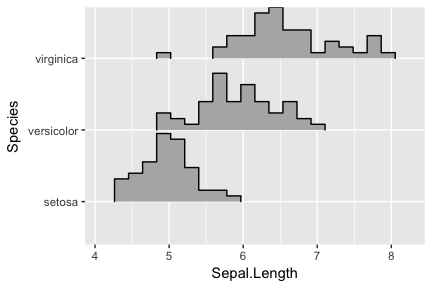# Introduction to ggjoy

#### 2017-07-30

Joyplots are partially overlapping line plots that create the impression of a mountain range. They can be quite useful for visualizing changes in distributions over time or space. The name “joyplot” was proposed by Jenny Bryan on Twitter on April 24, 2017, in reference to the iconic cover art for Joy Division’s album Unknown Pleasures.

## Geoms

The ggjoy package provides two main geoms, geom_ridgeline and geom_joy. The former takes height values directly to draw ridgelines, and the latter first estimates data densities and then draws those using ridgelines.

### Ridgelines

The geom geom_ridgeline can be used to draw lines with a filled area underneath.

library(ggplot2)
library(ggjoy)

data <- data.frame(x = 1:5, y = rep(1, 5), height = c(0, 1, 3, 4, 2))
ggplot(data, aes(x, y, height = height)) + geom_ridgeline()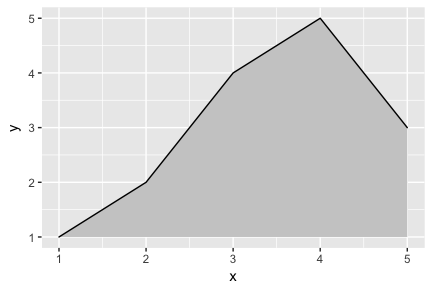Negative heights are allowed, but are cut off unless the min_height parameter is set negative as well.

# for side-by-side plotting
library(cowplot); theme_set(theme_gray())

data <- data.frame(x = 1:5, y = rep(1, 5), height = c(0, 1, -1, 3, 2))
plot_base <- ggplot(data, aes(x, y, height = height))
plot_grid(plot_base + geom_ridgeline(),
plot_base + geom_ridgeline(min_height = -2))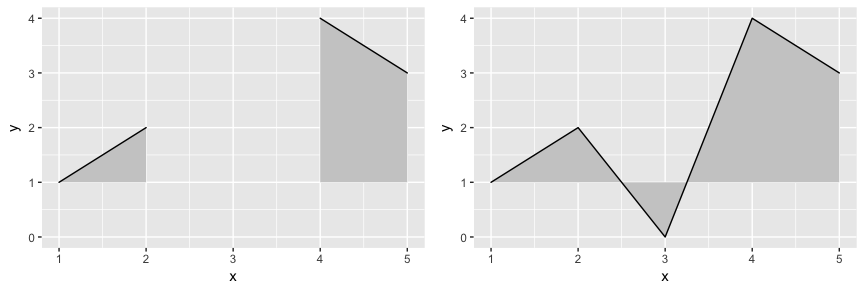Multiple ridgelines can be drawn at the same time. They will be ordered such that the ones drawn higher up are in the background. When drawing multiple ridgelines at once, the group aesthetic must be specified so that the geom knows which parts of the data belong to which ridgeline.

d <- data.frame(x = rep(1:5, 3), y = c(rep(0, 5), rep(1, 5), rep(2, 5)),
height = c(0, 1, 3, 4, 0, 1, 2, 3, 5, 4, 0, 5, 4, 4, 1))
ggplot(d, aes(x, y, height = height, group = y)) + geom_ridgeline(fill = "lightblue")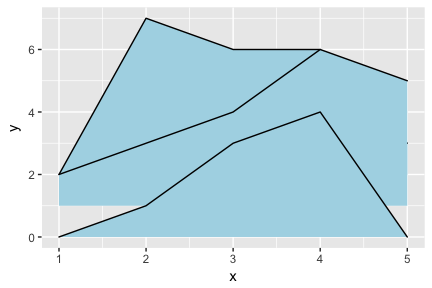It is also possible to draw ridgelines with geom_joy if we set stat="identity". In this case, the heights are automatically scaled such that the highest ridgeline just touches the one above at scale=1.

ggplot(d, aes(x, y, height = height, group = y)) +
geom_joy(stat = "identity", scale = 1)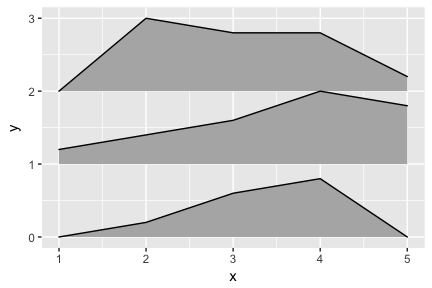### Density joyplots

The geom geom_joy calculates density estimates from the provided data and then plots those, using the ridgeline visualization. The height aesthetic does not need to be specified in this case.

ggplot(iris, aes(x = Sepal.Length, y = Species)) + geom_joy()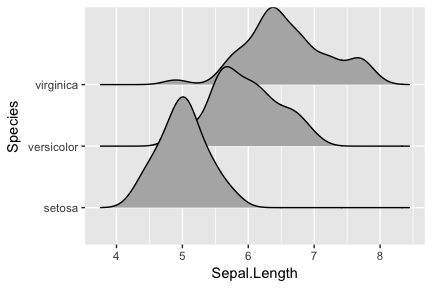There is also geom_joy2, which is identical to geom_joy except it uses closed polygons instead of ridgelines for drawing.

ggplot(iris, aes(x = Sepal.Length, y = Species)) + geom_joy2()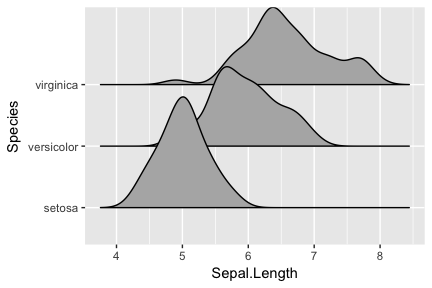The grouping aesthetic does not need to be provided if a categorical variable is mapped onto the y axis, but it does need to be provided if the variable is numerical.

# modified dataset that represents species as a number
iris_num <- transform(iris, Species_num = as.numeric(Species))

# does not work, causes error
# ggplot(iris_num, aes(x = Sepal.Length, y = Species)) + geom_joy()

# works
ggplot(iris_num, aes(x = Sepal.Length, y = Species_num, group = Species_num)) + geom_joy()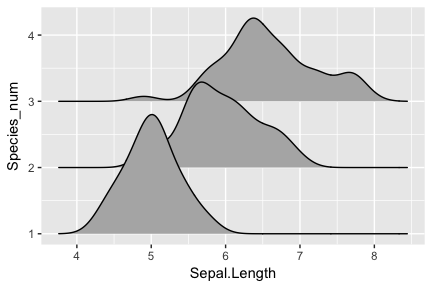Trailing tails can be cut off using the rel_min_height aesthetic. This aesthetic sets a percent cutoff relative to the highest point of any of the density curves. A value of 0.01 usually works well, but you may have to modify this parameter for different datasets.

ggplot(iris, aes(x = Sepal.Length, y = Species)) + geom_joy(rel_min_height = 0.01)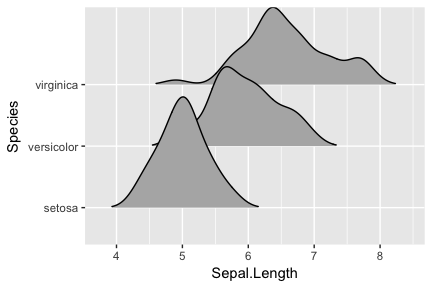The extent to which the different densities overlap can be controlled with the scale parameter. A setting of scale=1 means the tallest density curve just touches the baseline of the next higher one. Smaller values create a separation between the curves, and larger values create more overlap.

# scale = 0.9, not quite touching
ggplot(iris, aes(x = Sepal.Length, y = Species)) + geom_joy(scale = 0.9)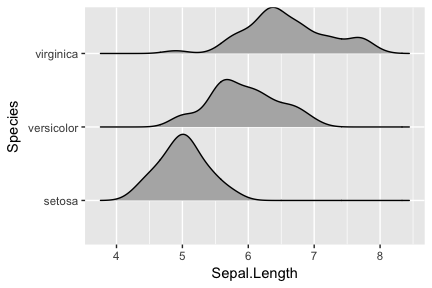# scale = 1, exactly touching
ggplot(iris, aes(x = Sepal.Length, y = Species)) + geom_joy(scale = 1)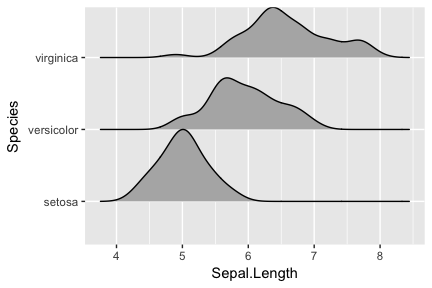# scale = 5, substantial overlap
ggplot(iris, aes(x = Sepal.Length, y = Species)) + geom_joy(scale = 5)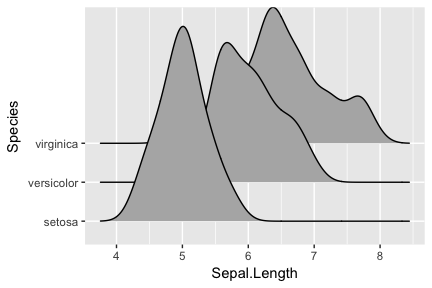The scaling is calculated separately per panel, so if we facet-wrap by species each density curve exactly touches the next higher baseline. (This can be disabled by setting panel_scaling = FALSE.)

ggplot(iris, aes(x = Sepal.Length, y = Species)) +
geom_joy(scale = 1) + facet_wrap(~Species)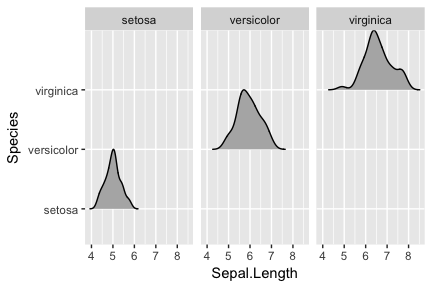## Themes

Joyplots tend to require some theme modifications to look good. Most importantly, the y-axis tick labels should be vertically aligned so that they are flush with the axis ticks rather than vertically centered. The ggjoy package provides a theme theme_joy that does this and a few other theme modifications.

ggplot(iris, aes(x = Sepal.Length, y = Species)) + geom_joy() + theme_joy()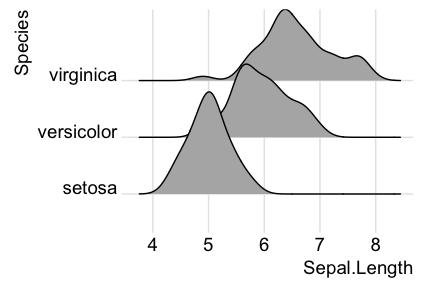However, without any further modifications, there are still a few issues with this plot. First, the ridgeline for the virginica species is slightly cut off at the very top point. Second, the space between the x and y axis labels and the ridgelines is too large. We can fix both issues using the expand option for the axis scales.

ggplot(iris, aes(x = Sepal.Length, y = Species)) +
geom_joy() + theme_joy() +
scale_x_continuous(expand = c(0.01, 0)) +
scale_y_discrete(expand = c(0.01, 0))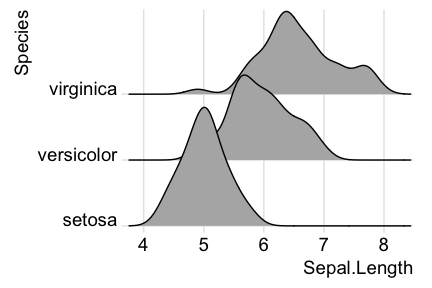By default, theme_joy adds a grid, but the grid can be switched off when not needed.

ggplot(iris, aes(x = Sepal.Length, y = Species)) +
geom_joy() + theme_joy(grid = FALSE) +
scale_x_continuous(expand = c(0.01, 0)) +
scale_y_discrete(expand = c(0.01, 0))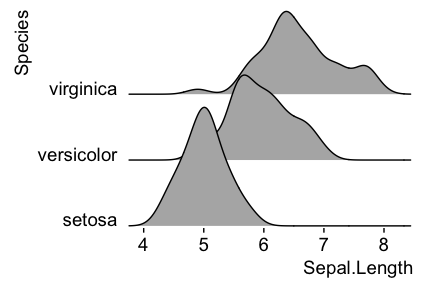If you prefer to use a different theme than theme_joy, for example theme_minimal, it is still advisable to adjust the alignment of the axis tick labels and the axis scales.

 ggplot(iris, aes(x = Sepal.Length, y = Species)) +
geom_joy() +
theme_minimal(base_size = 14) + theme(axis.text.y = element_text(vjust = 0)) +
scale_x_continuous(expand = c(0.01, 0)) +
scale_y_discrete(expand = c(0.01, 0))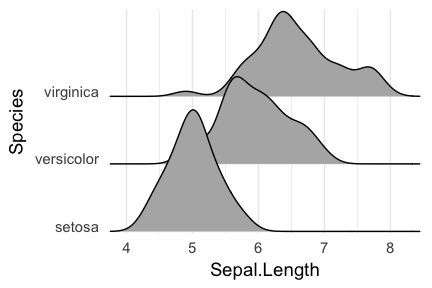## Cyclical scales

Many joyplots improve in appearance if the filled areas are drawn with alternating colors. To simplify the generation of such plots, ggjoy provides cyclical scales. These are scales that cycle through the aesthetic values provided. For example, if we use scale_fill_cyclical(values = c("blue", "green")) then ggplot will cycle through these two fill colors throughout the plot.

 ggplot(diamonds, aes(x = price, y = cut, fill = cut)) +
geom_joy(scale = 4) +
scale_fill_cyclical(values = c("blue", "green"))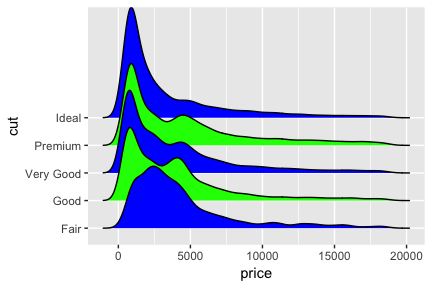By default, the cyclical scales will not draw a legend, because the legend will usually be confusing unless the labels are manually altered. Legends can be switched on via the guide = "legend" option, just like for all other scales.

 ggplot(diamonds, aes(x = price, y = cut, fill = cut)) +
geom_joy(scale = 4) +
scale_fill_cyclical(values = c("blue", "green"), guide = "legend")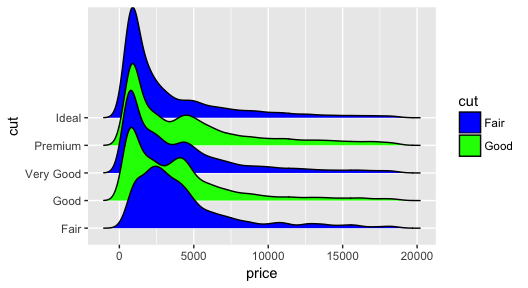Legends can be modified as usual.

 ggplot(diamonds, aes(x = price, y = cut, fill = cut)) +
geom_joy(scale = 4) +
scale_fill_cyclical(values = c("blue", "green"), guide = "legend",
labels = c("Fair" = "blue", "Good" = "green"),
name = "Fill colors")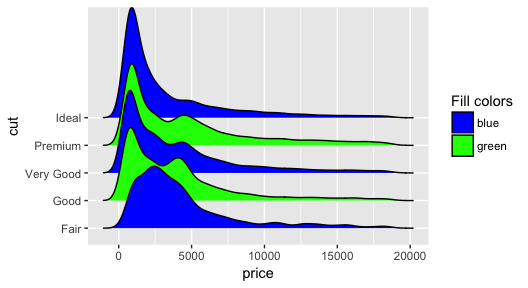Cyclical scales are defined for all the common aesthetics one might want to change, such as color, size, alpha, and linetype, and the legends are combined when possible

 ggplot(diamonds, aes(x = price, y = cut, fill = cut, color = cut)) +
geom_joy(scale = 4, size = 1) +
scale_fill_cyclical(values = c("blue", "green"), guide = "legend",
labels = c("Fair" = "blue w/ black outline",
"Good" = "green w/ yellow outline"),
name = "Color scheme") +
scale_color_cyclical(values = c("black", "yellow"), guide = "legend",
labels = c("Fair" = "blue w/ black outline",
"Good" = "green w/ yellow outline"),
name = "Color scheme")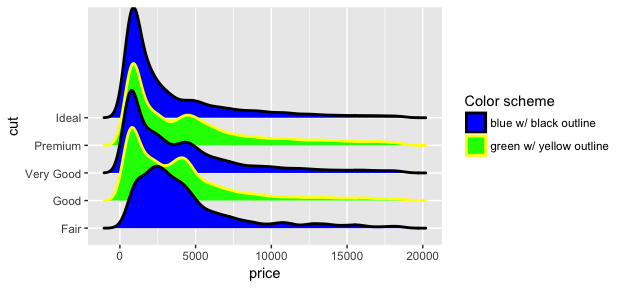Because these cyclical scales are generic ggplot2 scales, they work with any geom that accepts the respective aesthetic. Thus, for example, we can make histograms with alternatingly colored bars.

ggplot(mpg, aes(x = class, fill = class, color = class)) +
geom_bar(size = 1.5) +
scale_fill_cyclical(values = c("blue", "green"), guide = "legend",
labels = c("blue w/ black outline", "green w/ yellow outline"),
name = "Color scheme") +
scale_color_cyclical(values = c("black", "yellow"), guide = "legend",
labels = c("blue w/ black outline", "green w/ yellow outline"),
name = "Color scheme")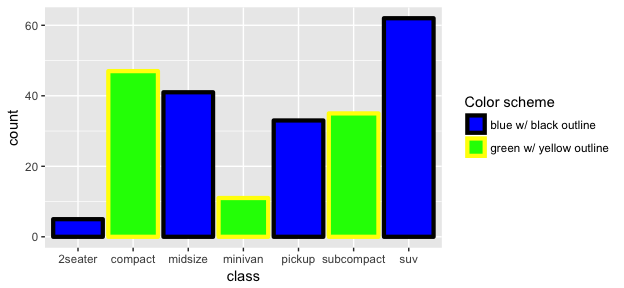While the previous example won’t win any design awards, more subtle effects can be helpful.

library(tidyverse)
mpg %>% group_by(class) %>% tally() %>% arrange(desc(n)) %>%
mutate(class = factor(class, levels=class)) %>%
ggplot(aes(x = class, y = n, fill = class)) +
geom_col() + theme_minimal() +
scale_fill_cyclical(values = c("#4040B0", "#9090F0")) +
scale_y_continuous(expand = c(0, 0))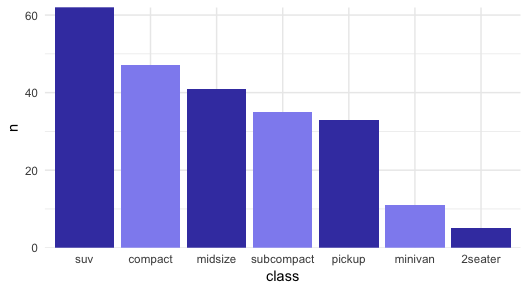## Stats

The default stat used with geom_joy is stat_joy. However, it may not do exactly what you want it to do, and there are other stats that can be used that may be better for your respective application.

First, stat_joy estimates the data range and bandwidth for the density estimation from the entire data at once, rather than from each individual group of data. This choice makes joyplots look more uniform, but the density estimates can in some cases look quite different from what you would get from geom_density or stat_density. This problem can be remidied by using stat_density with geom_joy. This works just fine, we just need to make sure that we map the calculated density onto the height aesthetic.

ggplot(iris, aes(x = Sepal.Length, y = Species, height = ..density..)) +
geom_joy(stat = "density")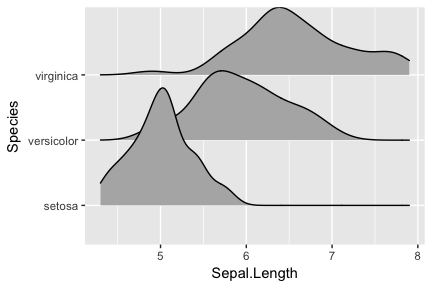Second, there may be scenarios in which you don’t want geom_joy to do any density estimation, for example because you have done so already yourself. In this case, you can use stat_identity. The benefit of using geom_joy with stat_identiy over using geom_ridgeline directly is that geom_joy provides automatic scaling.

As an example, assume we have calculated density curves for the Sepal.Length column in the iris dataset:

library(tidyverse)
iris %>% group_by(Species) %>%
do(ggplot2:::compute_density(.\$Sepal.Length, NULL)) %>%
rename(Sepal.Length = x) -> iris_densities
head(iris_densities)
## # A tibble: 6 x 6
## # Groups:   Species 
##   Species Sepal.Length      density       scaled      count     n
##    <fctr>        <dbl>        <dbl>        <dbl>      <dbl> <int>
## 1  setosa     3.931426 0.0008691193 0.0007010334 0.04345596    50
## 2  setosa     3.935804 0.0009731303 0.0007849289 0.04865652    50
## 3  setosa     3.940182 0.0010860774 0.0008760323 0.05430387    50
## 4  setosa     3.944560 0.0012082120 0.0009745463 0.06041060    50
## 5  setosa     3.948938 0.0013465664 0.0010861432 0.06732832    50
## 6  setosa     3.953316 0.0014984890 0.0012086843 0.07492445    50

We can plot these as follows:

ggplot(iris_densities, aes(x = Sepal.Length, y = Species, height = density)) +
geom_joy(stat = "identity")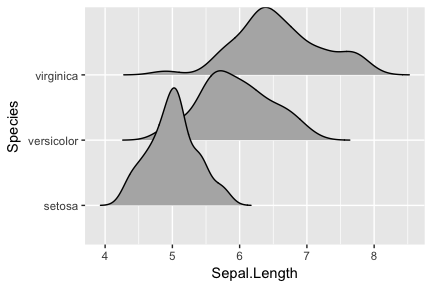Notice how this plot looks different from the one generated using stat = "density", even though the density computation was exactly the same: (i) The density curves extend all the way to zero. (ii) There is no horizontal line extending all the way to the limits of the x axis.

Finally, if you prefer histograms to density plots, you can also use stat_binline. Note that overlapping histograms can look strange, so this option is probably best used with a scale parameter < 1. The option draw_baseline = FALSE removes trailing lines to either side of the histogram. (For histograms, the rel_min_height parameter doesn’t work very well.)

ggplot(iris, aes(x = Sepal.Length, y = Species, height = ..density..)) +
geom_joy(stat = "binline", bins = 20, scale = 0.95, draw_baseline = FALSE)